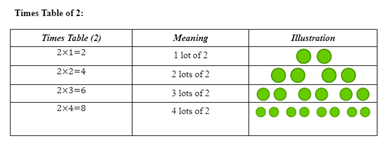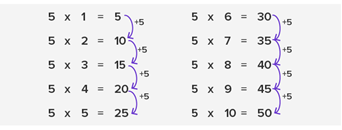# Multiplication Tables Worksheets

What Are Times Tables? A times table is largely associated with multiplication. It is the multiplication calculations for all the numbers starting from 1 x 2 to 12 x 10. Children can begin to master the art of time table by learning the 2x, 5x, and 10x tables. It is a list of multiples of a particular number. For instance, if you want to work out the times-table for 2, you will be required to start with 2 and then keep adding 2 in each step as you move along the table. The answers you will get in every step will be a multiple of 2 and known as multiplication fact. Take a look at the following times tables illustrated below:There is more than one way of working out the times-table. You can try out different methods of doing it. Another way to work it out is given below.• ### Basic Lesson

Introduces the concept of Multiplication Tables.

• ### Independent Practice 1

Students practice with 12 Multiplication Tables problems. The answers can be found below. Fill in the missing numbers in the multiplication tables.

• ### Independent Practice 2

Another 12 time Multiplication Tables problems. The answers can be found below.

• ### Homework Worksheet

Reviews all skills in the unit. A great take home sheet. Also provides a practice problem.

• ### Skill Quiz

10 problems that test times table skills.

• ### Homework and Quiz Answer Key

Answers for the homework and quiz.